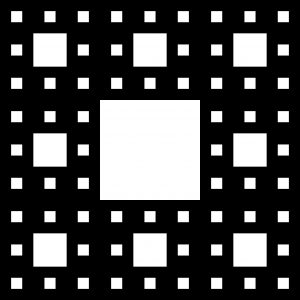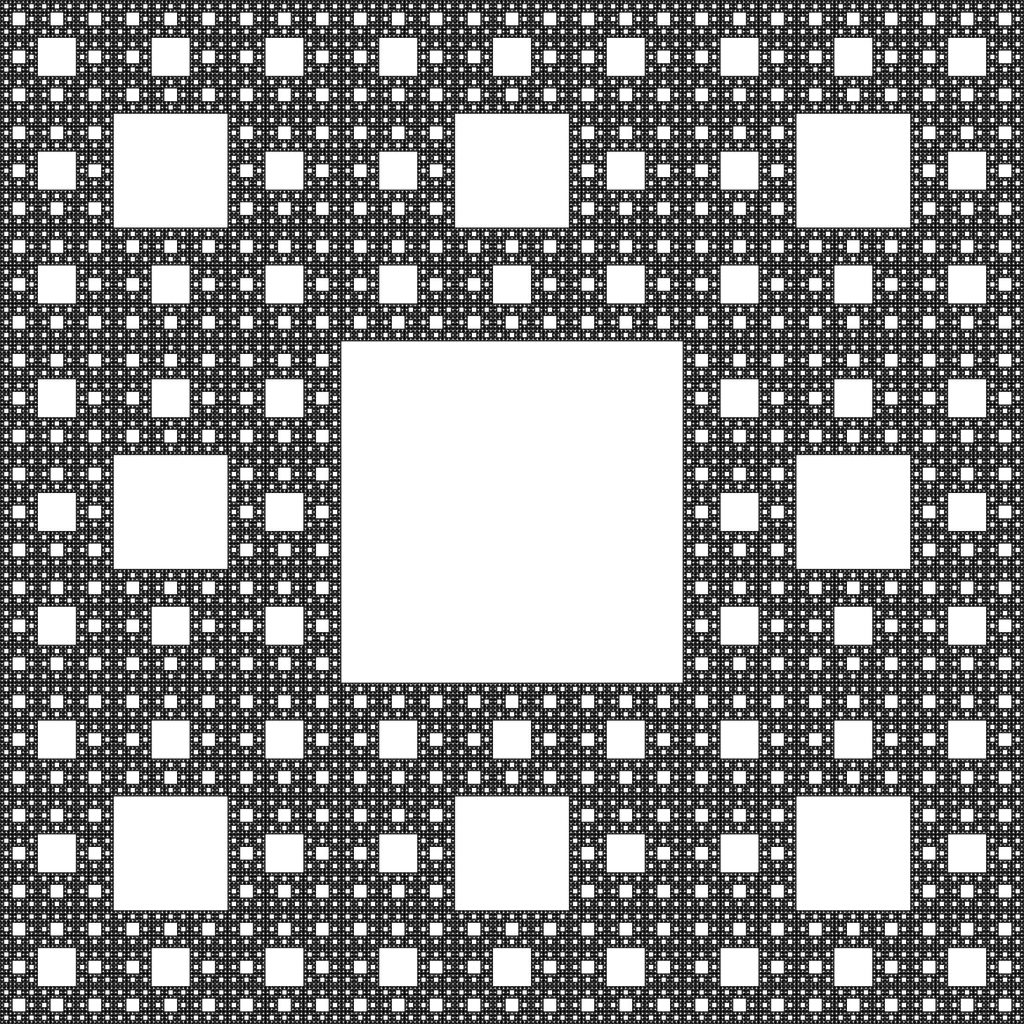# Python | Sierpinski Carpet

• Difficulty Level : Easy
• Last Updated : 06 Nov, 2018

The Sierpinski Carpet is a plane fractal curve i.e. a curve that is homeomorphic to a subspace of plane. It was first described by Waclaw Sierpinski in 1916. In these type of fractals, a shape is divided into a smaller copy of itself, removing some of the new copies and leaving the remaining copies in specific order to form new shapes of fractals.

How is it constructed?
The Sierpinski Carpet starts with a square. This square is divided into nine equal parts. The centremost smaller square is removed from the original larger square. The remaining square pieces are then again divided into nine equal parts and the centermost square from each square is removed. On repeating this process, a beautiful pattern of Sierpinski Carpet is observed.

Attention geek! Strengthen your foundations with the Python Programming Foundation Course and learn the basics.

To begin with, your interview preparations Enhance your Data Structures concepts with the Python DS Course. And to begin with your Machine Learning Journey, join the Machine Learning - Basic Level CourseWe divide it into 9 equal pieces and remove the center square.On further repeating the process, it results in something like this.We can visualize this phenomenon in details in this video.

Let us see what its code looks like :

 `# importing necessary modules``import` `numpy as np``from` `PIL ``import` `Image`` ` `# total number of times the process will be repeated``total ``=` `7`` ` `# size of the image``size ``=` `3``*``*``total`` ` `# creating an image``square ``=` `np.empty([size, size, ``3``], dtype ``=` `np.uint8)``color ``=` `np.array([``255``, ``255``, ``255``], dtype ``=` `np.uint8)`` ` `# filling it black``square.fill(``0``)`` ` `for` `i ``in` `range``(``0``, total ``+` `1``):``    ``stepdown ``=` `3``*``*``(total ``-` `i)``    ``for` `x ``in` `range``(``0``, ``3``*``*``i):``         ` `        ``# checking for the centremost square``        ``if` `x ``%` `3` `=``=` `1``:``            ``for` `y ``in` `range``(``0``, ``3``*``*``i):``                ``if` `y ``%` `3` `=``=` `1``:``                     ` `                    ``# changing its color``                    ``square[y ``*` `stepdown:(y ``+` `1``)``*``stepdown, x ``*` `stepdown:(x ``+` `1``)``*``stepdown] ``=` `color`` ` `# saving the image produced``save_file ``=` `"sierpinski.jpg"``Image.fromarray(square).save(save_file)`` ` `# displaying it in console``i ``=` `Image.``open``(``"sierpinski.jpg"``)``i.show()`

Output :This is the Sierpinski Carpet after 7 repetitions. You can get its code for other languages on rosettacode.

My Personal Notes arrow_drop_up